CBSE Class 10 Sample Paper for 2021 Boards - Maths Basic

Class 10
Solutions of Sample Papers for Class 10 Boards

## Due to heavy storm an electric wire got bent as shown in the figure. It followed a mathematical shape. Answer the following questions below.

Note: Check more Case Based Questions for Polynomials Class 10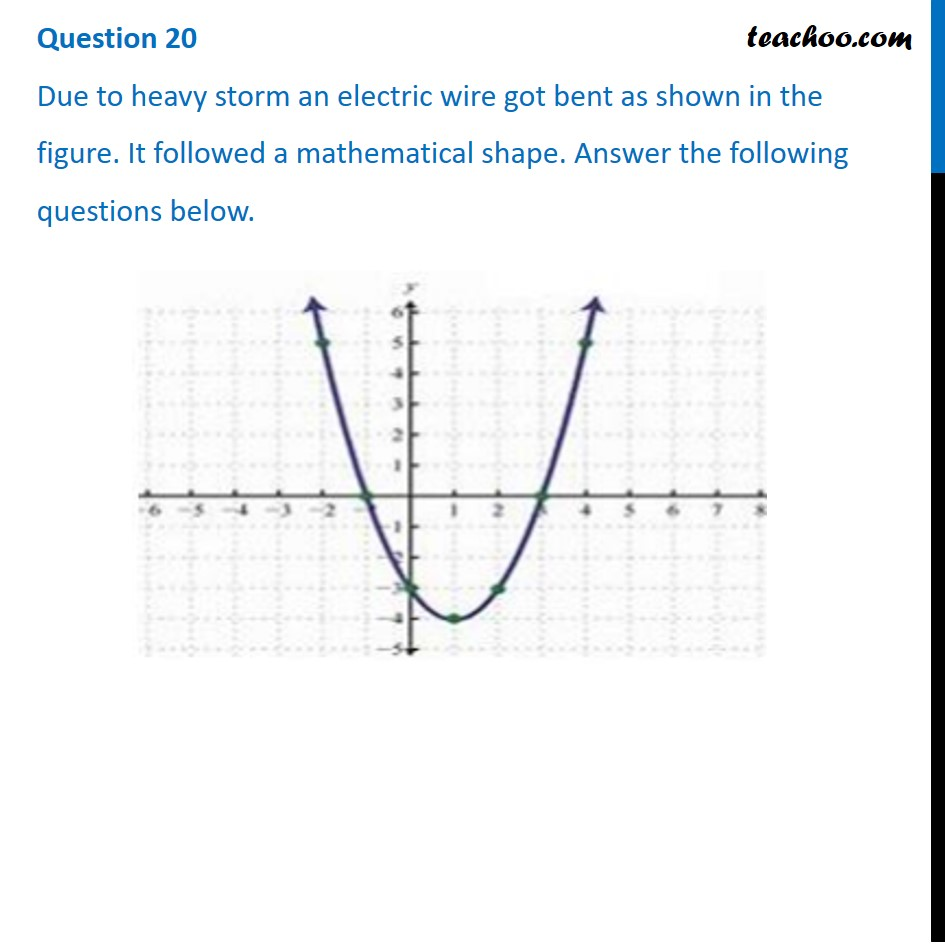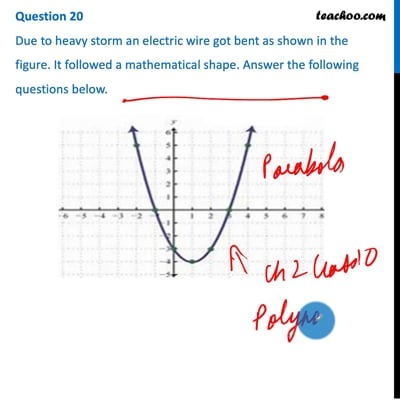This video is only available for Teachoo black users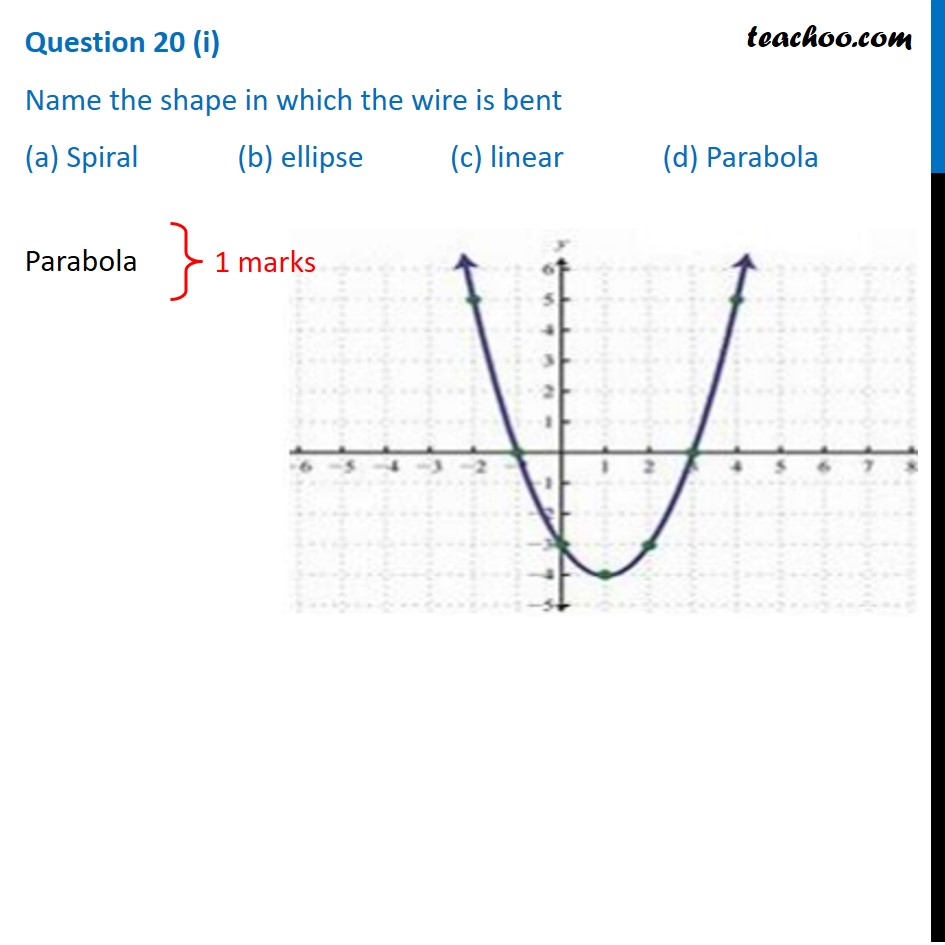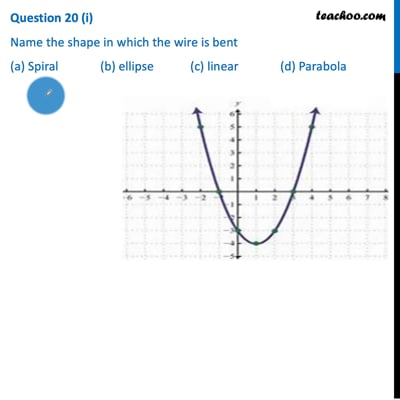This video is only available for Teachoo black users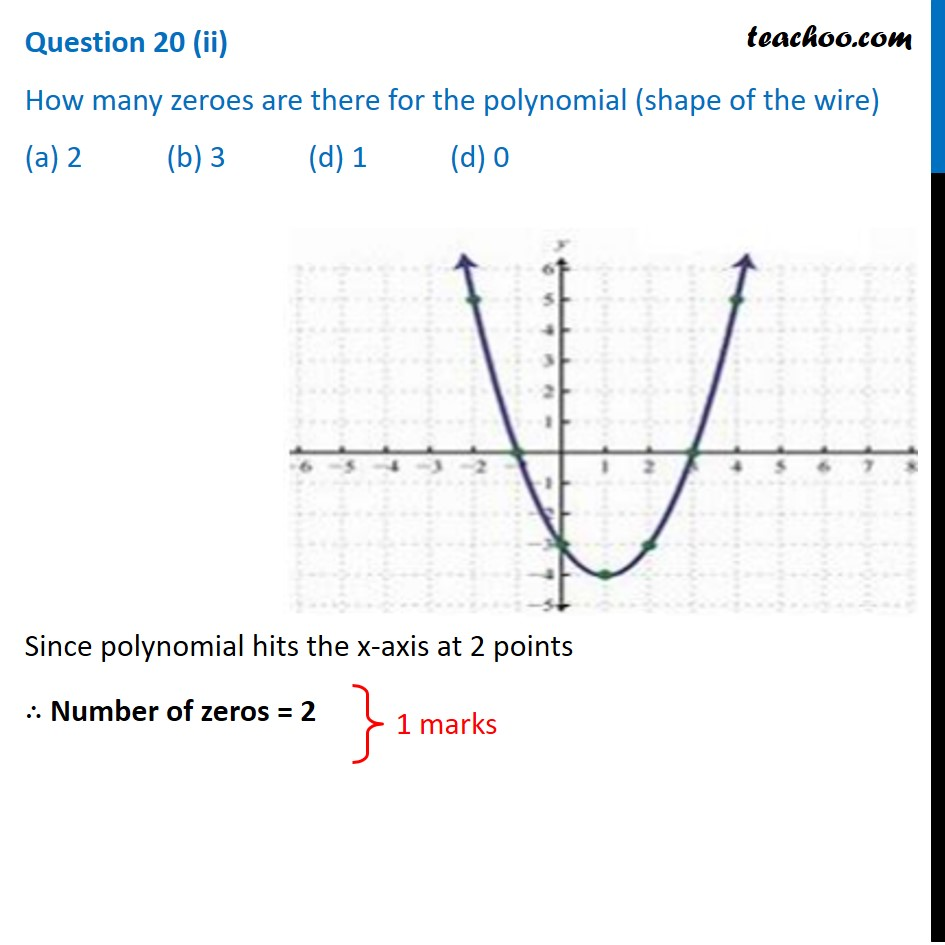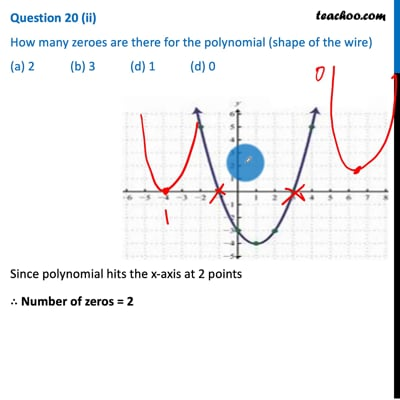This video is only available for Teachoo black users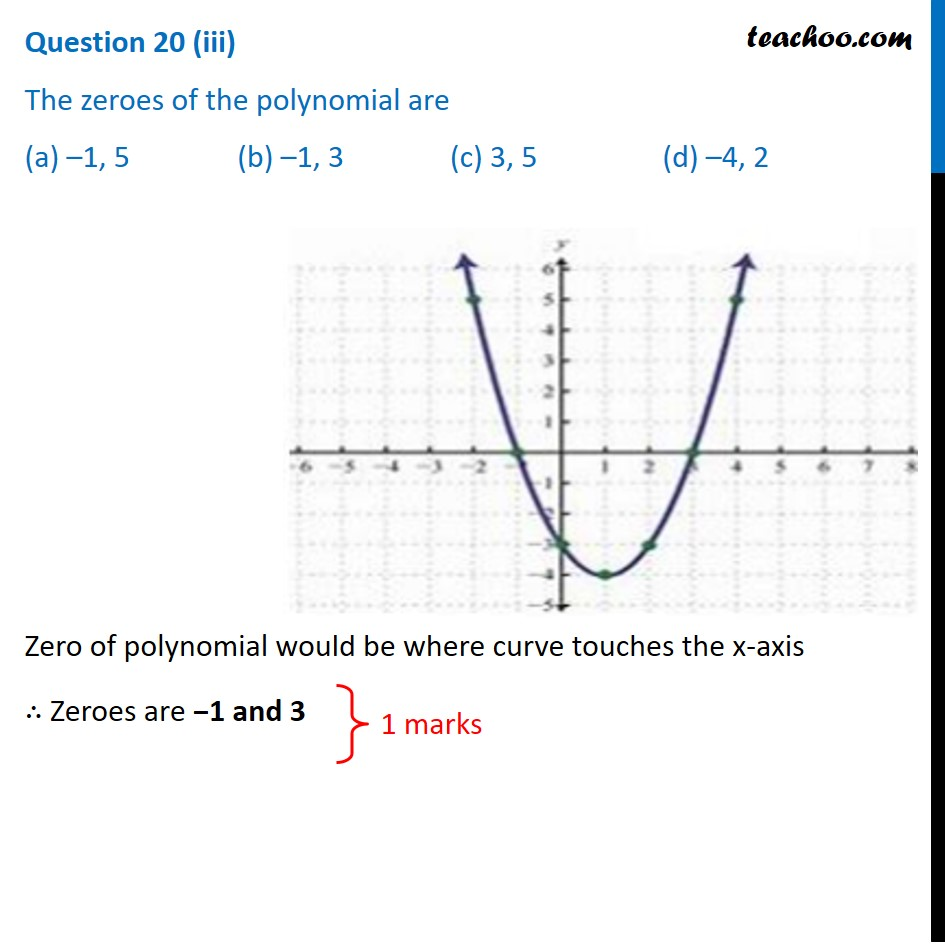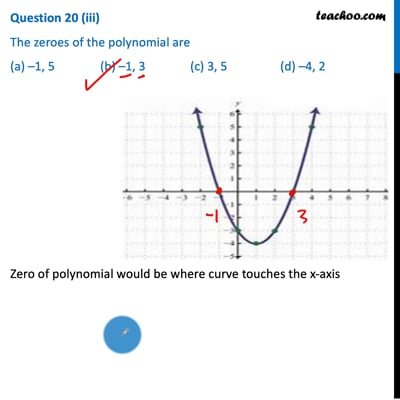This video is only available for Teachoo black users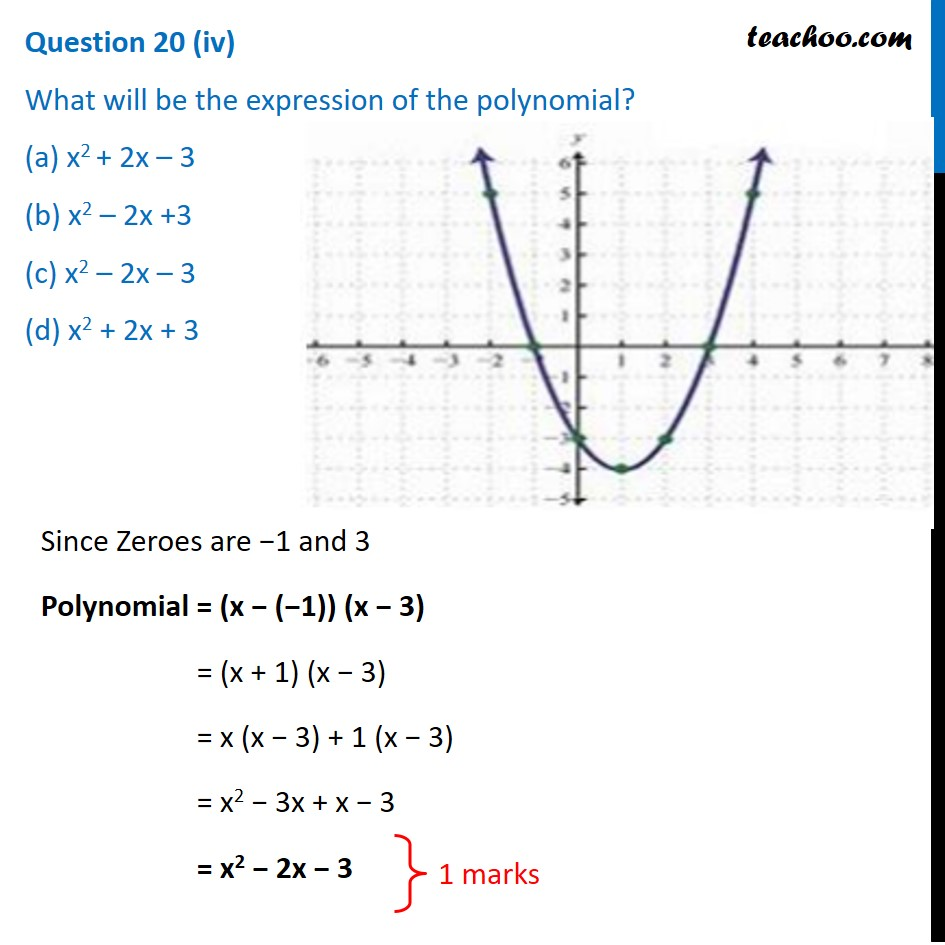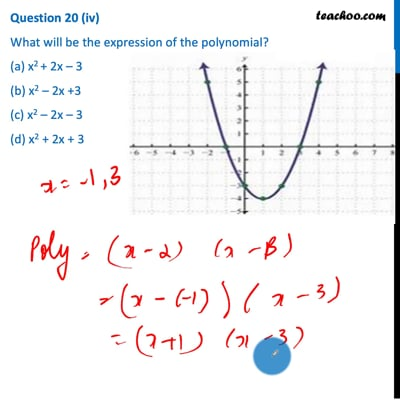This video is only available for Teachoo black users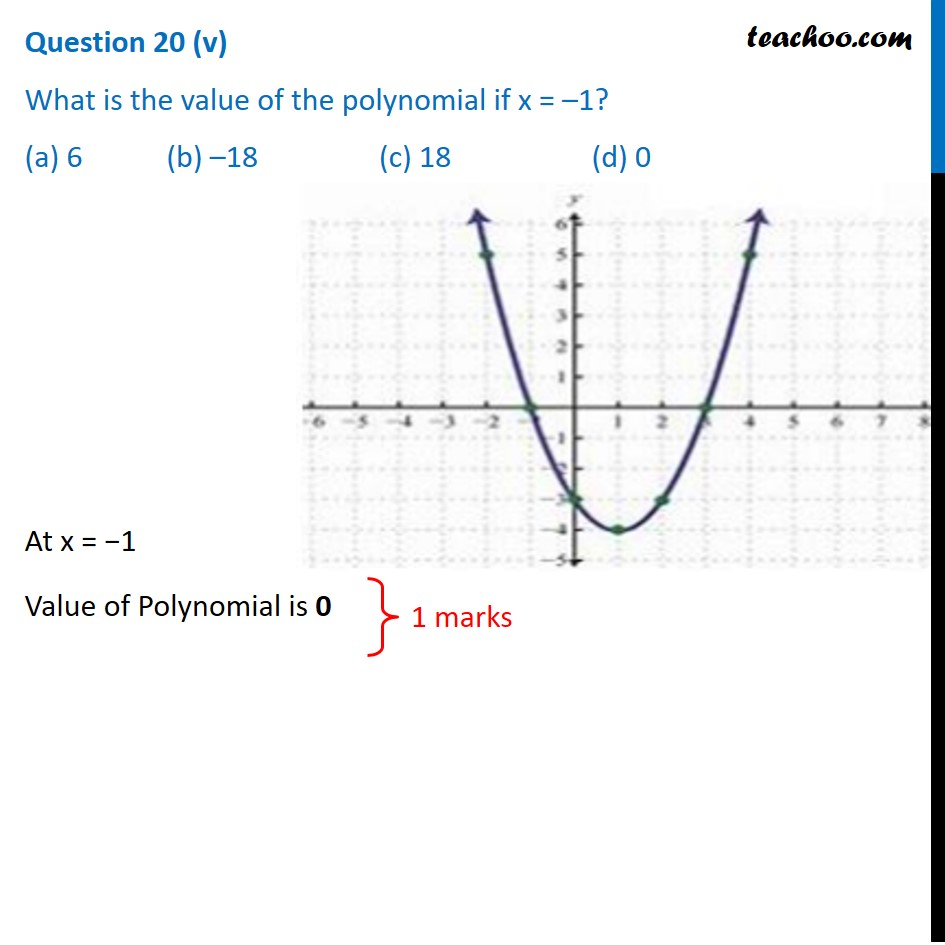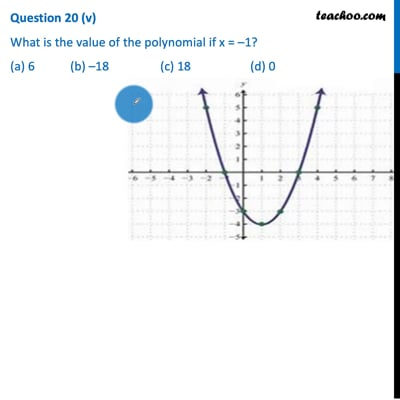This video is only available for Teachoo black users

Note: Check more Case Based Questions for Polynomials Class 10

### Transcript

Question 20 Due to heavy storm an electric wire got bent as shown in the figure. It followed a mathematical shape. Answer the following questions below.Question 20 (i) Name the shape in which the wire is bent (a) Spiral (b) ellipse (c) linear (d) ParabolaParabola 1 marks Question 20 (ii) How many zeroes are there for the polynomial (shape of the wire) (a) 2 (b) 3 (d) 1 (d) 0Since polynomial hits the x-axis at 2 points ∴ Number of zeros = 2 1 marks Question 20 (iii) The zeroes of the polynomial are (a) –1, 5 (b) –1, 3 (c) 3, 5 (d) –4, 2Zero of polynomial would be where curve touches the x-axis ∴ Zeroes are −1 and 3 1 marks Question 20 (iv) What will be the expression of the polynomial? (a) x2 + 2x – 3 (b) x2 – 2x +3 (c) x2 – 2x – 3 (d) x2 + 2x + 3Since Zeroes are −1 and 3 Polynomial = (x − (−1)) (x − 3) = (x + 1) (x − 3) = x (x − 3) + 1 (x − 3) = x2 − 3x + x − 3 = x2 − 2x − 3 1 marks Question 20 (v) What is the value of the polynomial if x = –1? (a) 6 (b) –18 (c) 18 (d) 0At x = −1 Value of Polynomial is 0 1 marks